This article was published as a part of the Data Science Blogathon

## Introduction

So, In this article, we will discuss another approach, named matrix factorization to understand the LDA which is similar to that of Singular Value Decomposition (SVD) which we discussed in our previous article.

This is part-19 of the blog series on the Step by Step Guide to Natural Language Processing.

1. Matrix Factorization Approach for LDA

2. Parameters involved in LDA

4. Tips to improve results of Topic Modelling using LDA

## Matrix Factorization approach for LDA

Let’ see the step-by-step procedure of the matrix factorization approach for LDA.

### Step-1

Create a document term matrix that shows a corpus of N documents D1, D2, D3 … Dn and vocabulary size of M words W1, W2 .. Wn. In that matrix, a particular cell (i, j) represents the frequency count of word Wj in the Document Di of the corpus.

For Example, a sample matrix of the above is described below: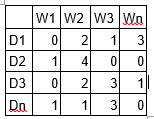### Step-2

LDA converts this Document-Term Matrix into two lower dimensional matrices, M1 and M2 where M1 and M2 represent the document-topics and topic–terms matrix with dimensions (N, K) and (K, M) respectively, where

• N is the number of documents,
• K is the number of topics,
• M is the vocabulary size.

For Example, A sample matrix M1 (document-topics) is described below: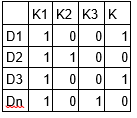Also, a sample matrix M2 (topic-terms) is described below: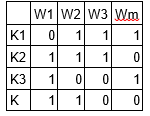Image Source: Google Images

Notice that two matrices M1 and M2 already provide topic word and document topic distributions. However, we have to improve these distributions, which is the main goal of LDA. So, LDA makes use of some sampling techniques in order to improve these matrices.

### Step-3

In this step, we iterate through each word “w” present in each of the document “d” and tries to adjust the current topic – word assignment with a new assignment.

A new topic “k” is assigned to the word “w” with a probability P which is the multiplication of two probabilities p1 and p2.

### Step-4

For every topic, the following two probabilities p1 and p2 are calculated.

• p1: p(topic t / document d) represents the proportion of words in document d that are currently assigned to topic t.
• p2: p(word w / topic t) represents the proportion of assignments to topic t over all documents that come from this word w.

### Step-5

The current topic – word assignment is updated with a new topic with the probability, which is the product of p1 and p2 probabilities.

In this step, the model assumes that all the existing word–topic assignments except the current word are correct. This is essentially the probability that topic t generated word w, so it makes sense to adjust the current word’s topic with a new probability.

### Step-6

After a number of iterations, we achieved a steady-state where the document topic and topic term distributions are fairly good and This is considered as the convergence point for LDA.

## Visualization

In the previous article on the probabilistic approach on LDA, we derived that P(w|d) is equal to: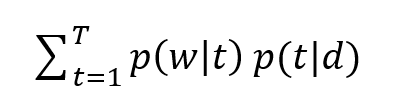i.e., represents the dot product of ϴtd and Фwt for each topic t.

The above thing can be also represented in the form of a matrix (shown below):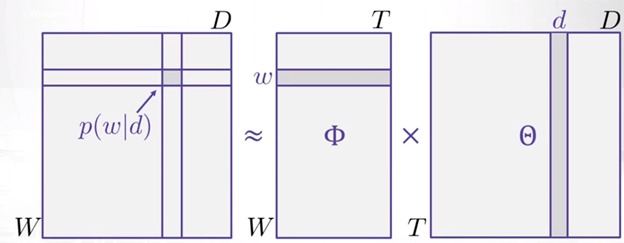Image Source: Google Images

So, by looking at the above diagram, we can think of LDA similar to that of matrix factorization or SVD, where we decompose the probability distribution matrix of the word in the document in two matrices consisting of distribution of topic in a document and distribution of words in a topic.

LDA factorizes the Document-term matrix into how many matrices?

• 1
• 2
• 3
• 4

## Parameters Involved in LDA

Following are the parameters involved while implementing LDA are as follows:

### Alpha and Beta Hyperparameters

Alpha and beta represent the document-topic density and topic-word density respectively.

Interpretation of Alpha:

The ‘α’ hyperparameter controls the number of topics expected in the document.

Higher the value of alpha, we expect that documents are composed of more topics in the mixture, and lower the value of alpha, we expect that documents contain fewer topics in the mixture.

Interpretation of Beta:

The ‘β’ hyperparameter controls the distribution of words per topic.

At lower values of ‘β’, the topics will be composed of fewer words, and at higher values of beta,  the topics will be composed of a large number of words in the corpus.

### What values of alpha and beta one should expect ideally?

Ideally, we would want to see only a few topics in each document and also few words in each of the topics. So, we typically set the values of α and β less than one.

1. In LDA, which of the following parameter represents document-topic density?

• Beta
• Alpha
• Delta
• Gamma

2. In LDA, which of the following parameter represents topic-word density?

• Beta
• Alpha
• Delta
• Gamma

### Number of Topics

This parameter represents how many topics you want to be extracted from the corpus.

### How to choose the value of hyperparameter ‘K’?

The ‘K’ hyperparameter specifies the number of topics one should expect from the corpus of documents. Generally, while we choose the value of K, we require domain knowledge. But some researchers have developed different approaches to obtain an optimal number of topics such as,

1. Kullback Leibler Divergence Score.

2. An alternate way is to train different LDA models with different numbers of K values and compute the ‘Coherence Score’ and then choose that value of K for which the coherence score is highest.

### Number of Topic Terms

This parameter represents how many terms composed in a single topic.

### How to decide the number of topic terms?

It is generally decided according to the requirement.

If the problem statement deals with extracting themes or concepts, it is recommended to choose a higher number, otherwise, if the problem statement deals with extracting features or terms, a low number is recommended.

### Number of Iterations or passes

The maximum number of iterations allowed to LDA algorithm for convergence.

Some of the advantages of LDA are as follows:

### Fast

The model is usually fast to run. But of course, it depends on your data. You can verify it by using the %time command in Jupyter Notebook.

Several factors which can slow down the model are as follows:

• Very long documents
• A large number of documents in the corpus
• Large vocabulary size, especially when you use n-grams with a very high value of n

### Intuitive

This Modelling approach to extract the topics gives weighted lists of words which is a very simple approximation yet a very intuitive approach for interpretation, as there is no embedding nor hidden dimensions, just bags of words with corresponding weight values.

### Can predict topics for new unseen documents

Once your model is trained, it is ready to allocate topics to any document.

But the thing you have to remember that is if your training dataset is in English and you want to predict the topics of a Chinese document it will not work. But if the new documents have the same structure as that of the training dataset and should have more or less the same topics, it will work.

Some of the disadvantages of LDA are as follows:

### Requires Lots of fine-tuning

If LDA is fast to run, it will give you some trouble to get good results with it. That’s why knowing in advance how to fine-tune it will really help you.

### Needs Human Interpretation

After finding the Topics from the set of documents with the help of a machine, we also require manual human efforts to label them in order to present the results to non-experts people.

### You cannot influence topics

Sometimes what happens is that based on our prior knowledge we know some of the topics that your documents talk about, but when we do the same thing with the help of LDA, you will not find those topics, which will definitely be frustrating for you. And there is no method to say to the model that some words should belong together. So, you have to sit and wait for the LDA to give you what you want.

## Methods to Improve Results of LDA

The results of topic modelling algorithms are completely dependent on the features (terms) present in the corpus. As we know that while we represent our corpus with a document term matrix, generally we get a very sparse matrix.

So, to improve the results of these algorithms we can reduce the dimensionality of the matrix. Based on some experiments, there are few approaches that you can try to improve the results of topic modelling algorithms:

• Frequency Filter
• Part of Speech Tag Filter
• Batch Wise LDA

### Frequency Filter

The main idea behind this technique is to arrange every term according to its frequency.

We want terms with higher frequencies to appear more likely in the results as compared to ones with low frequency since the low-frequency terms are essentially the weak features of the corpus. Hence it is a good practice to get remove all those weak features present in the corpus.

To decide the threshold of frequency, you have to do an exploratory analysis of terms and their frequency.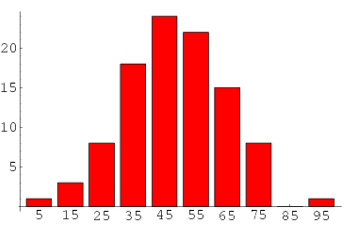### Part of Speech Tag Filter

POS tag filter is related more to the context of the features than the frequencies of features.

As we know that the topic Modelling tries to map out the recurring patterns of terms into topics. But while doing this in terms of context, every term might not be equally important.

For example, POS tag IN contains terms such as – “within”, “upon”, “except”. “CD” contains – “one”,” two”, “hundred” etc. “MD” contains “may”, “must” etc.

The above terms are the supporting words of a language and can be removed by studying their post tags.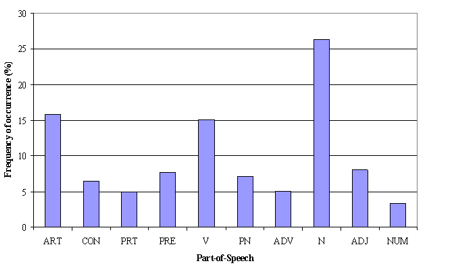### Batch Wise LDA

In order to retrieve the most important topic terms, a corpus can be divided into batches of fixed sizes. And then apply the LDA multiple times on these batches will provide different results. Finally, the best topic terms will be the intersection of all the batches.

NOTE: The techniques we described to improve the results of the topic model can apply to all the topic modeling algorithms, which means they are not restricted only to LDA. So, while you are working on an NLP task, you can play with these techniques and improve your results.

1. Less frequent terms present in the corpus adds noise in the topic assignment made by LDA.

• True
• False

2. Figure of Speech Tag Filter aid in improving the result of topic modelling.

• True
• False

## Other Blog Posts by Me

You can also check my previous blog posts.

Previous Data Science Blog posts.

Here is my Linkedin profile in case you want to connect with me. I’ll be happy to be connected with you.

## Email

For any queries, you can mail me on Gmail.

## End Notes